# Mathematics at Aksharam

Want to share an opinion?—drop a mail

Dr. Lalitha Subramanian, About the Author

# CBSE X Mathematics

## CHAPTER 2: Polynimials

### 2-1: Zeros of a Polynomial

We have studied inprevious classes about polynomials in one variable. We recall the important aspects below:

1. Standard form of writing a polynomial of one variable is $p(x) = a_{n}x^n + a_{n-1}x^{n-1} + ...... a_{2}x^2 + a_{1}x + a_{0}$ where $a_{n} \neq 0$.
2. Degree of the highest degree term in the polynomial is the degree of the polynomial.
3. If $k$ is any real number, then the value obtained by substituting $x = k$ in the polynomial is called the value of the polynomial at $x = k$ and denoted by $p(k)$.
4. If $p(k) = 0$ for some real number $k$, then $k$ is said to be the zero of the polynomial..

A polynomial of degree $1$ is called a linear polynomial. Standard form of a linear polynomial is $p(x) = ax + b$. Examples of a linear polynomial are $p(x) = 2x + 3$, $p(x) = -5x + \frac{2}{3}$, $p(x) = 2x$, etc. A linear polynomial has at most two terms.
A polynomial of degree $2$ is called a quadratic polynomial . Standard form of a quadratic polynomial is $p(x) = ax^2 + bx + c$. Examples include $p(x) = 2x^2 -7x + 4$, $p(x) = -x^2 + 4x$, $p(x) = 4x^2 - 9$, etc. A quadratic polynomal as at most three terms.
A cubic polynomial is one with degree $3$. Standard form of a cubic polynomial is $p(x) = ax^3 + bx^2 + cx + d$. Examples of cubic polynomial include $p(x) = 2x^3 + 5x^2 - x + 8$, $p(x) = -x^3 + 4x^2 + 6$, $p(x) = 4x^3 + x - 10$, $p(x) = -5x^3 + 7x^2 + x$, etc. A cubic polynomial has at most four terms.
In general, a polynomial of $n$ degree has at most $n+1$ terms.

Zeros of the polynomial can be obtained by setting $p(x) = 0$ and solving for $x$. For example, the zero of a linear polynomial $p(x) = 2x - 1$ is obtained by setting $2x - 1 = 0$, which gives $x = \frac{1}{2}$. In general zero of a linear polynomial $p(x) = ax + b$ is $x = -\frac{b}{a}$.

Geometrically, zero of a polynomial is the point where the graph of the polynomial $y = p(x)$ intersects the $x$-axis, which is also called the $x$- intercept. A polynomial of degree $n$ has at most $n$ $x$-intercepts.

#### Example 1:

Graph the linear polynomial $2x - 1$ and show the $x$- intercept. Verify that the zero of this polynomial is the $x$-intercept.

Solution:

The table below gives the values of $y$ for which $y = 2x -1$ . As two distinct points determine a unique line, it is sufficient to determine the coordinates of two points on this line.
\begin{array}{ccc} x & y=2x-1 & (x,y)\\ \hline 0 & -1 & (0,-1)\\ 1 & 1 & (1,1)\\ \hline \end{array}
The graph shows the line $y = 2x - 1$. From the graph, it is clear that the line intersects the $x$-axis at the point $(\frac{1}{2}, 0)$. Algebraically, we can solve the equation $2x - 1 = 0$ to get $x = \frac{1}{2}$, thus verifying that the zero of this polynomial is the $x$-intercept.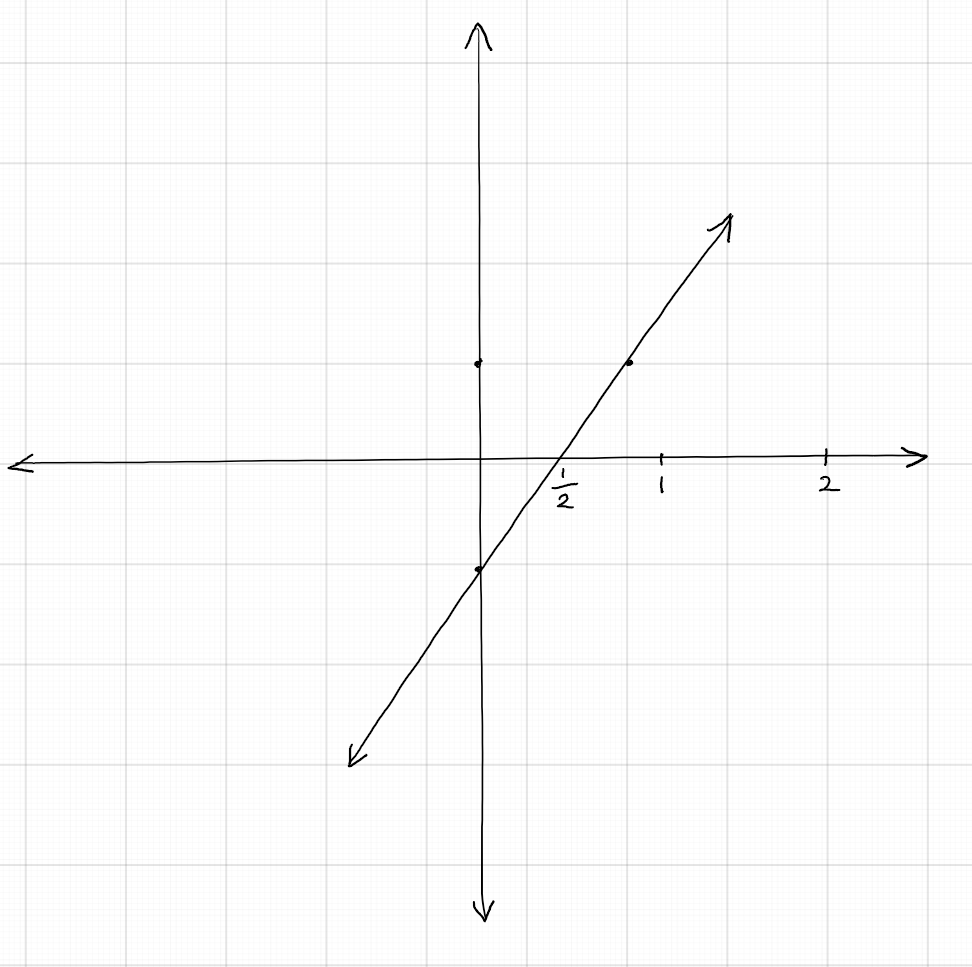In general, a linear polynomial has at most $1$ zero. This means that the graph has either $0$ or $1$ $x$-intercept. The example above shows the graph of a line that has $1$ $x$-intercept. The graph of a linear polynomial $y = ax + b$ is a rising line (going upward) is the leading coefficient $a \gt 0$ and a falling line (going downward) if the leading coefficient $a \lt 0$. A horizontal line has $0$ $x$-intercept. Equation of this line is $y = 0$. That is, it is a $0$ polynomial.

A quadratic polynomial has at most two zeros. That is, it can have $0$, $1$, or $2$ zeros. Geometrically, this means that the graph of a quadratic polynomial may have $0$, $1$, or $2$ $x$-intercepts. Algebraically, the zeros are obtained by solving the equation $ax^2 + bx + c = 0$. The following graphs are graphs of a quadratic polynomial with $0$, $1$, or $2$ zeros. Note that the graph is 'U' shaped, and opens upwards if the leading coefficient $a \gt 0$, and opens downwads when $a \lt 0$.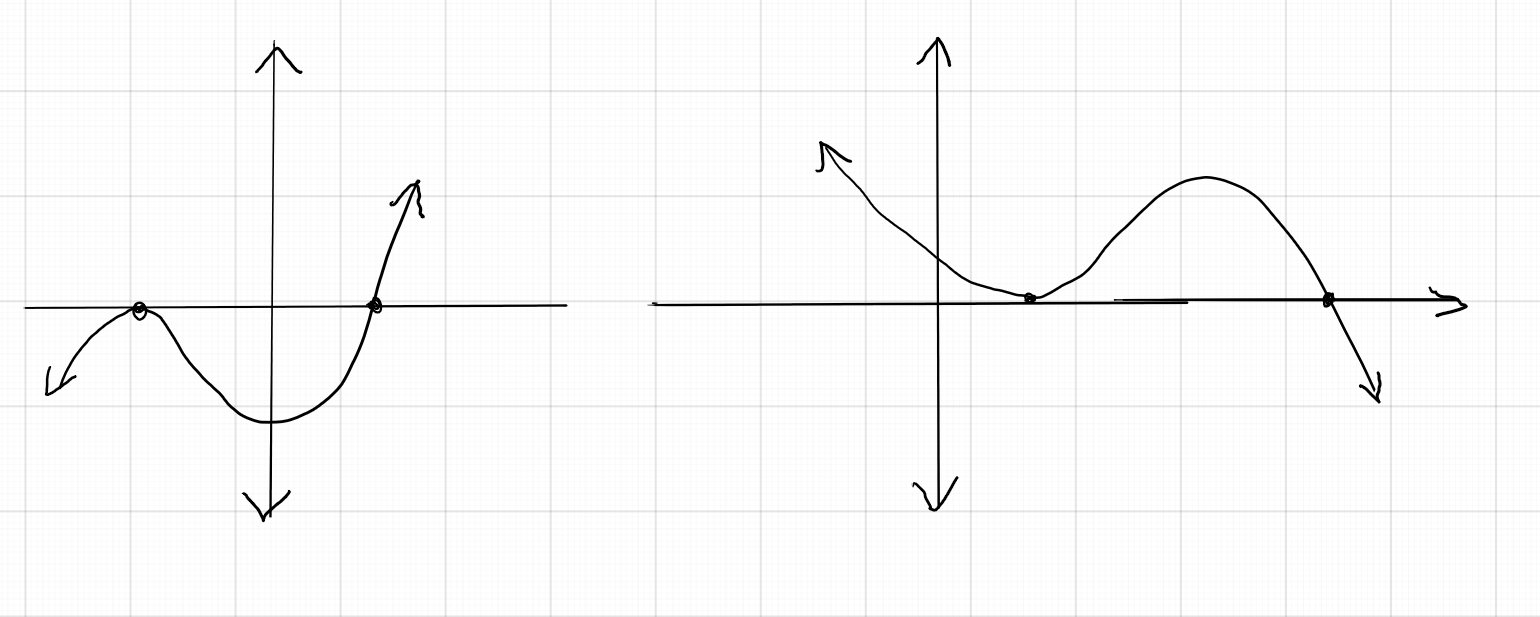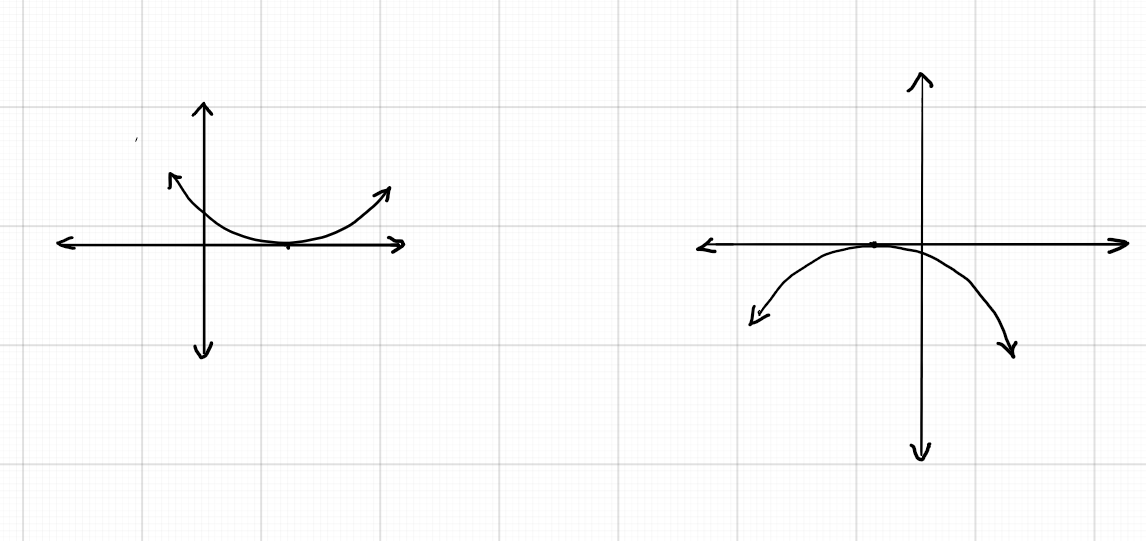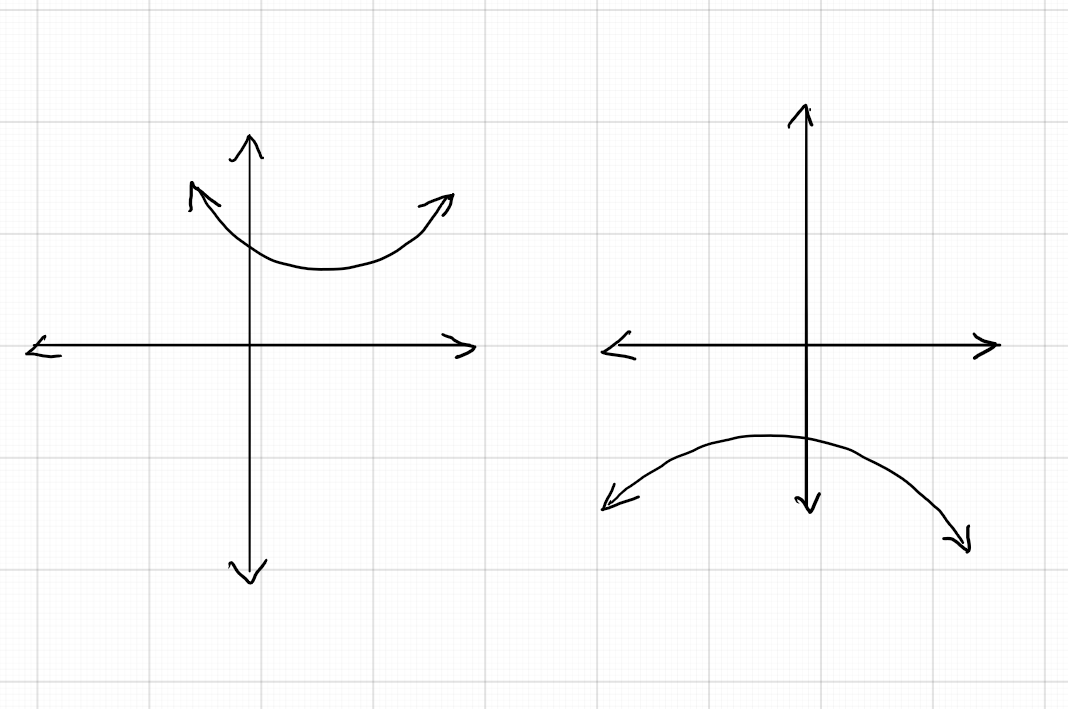A cubic polynomial has at most 3 zeros. That is, the graph of a cubic polynomial has $0$, $1$, $2$, or $3$ x-intercepts. The graph of a cubic polynomial starts going upward (rising) and takes 2 turns if the leading coefficient $a \gt 0$ and starts going downward (falling) and then takes two turns if $a \lt 0$. Following graphs illustrate this property.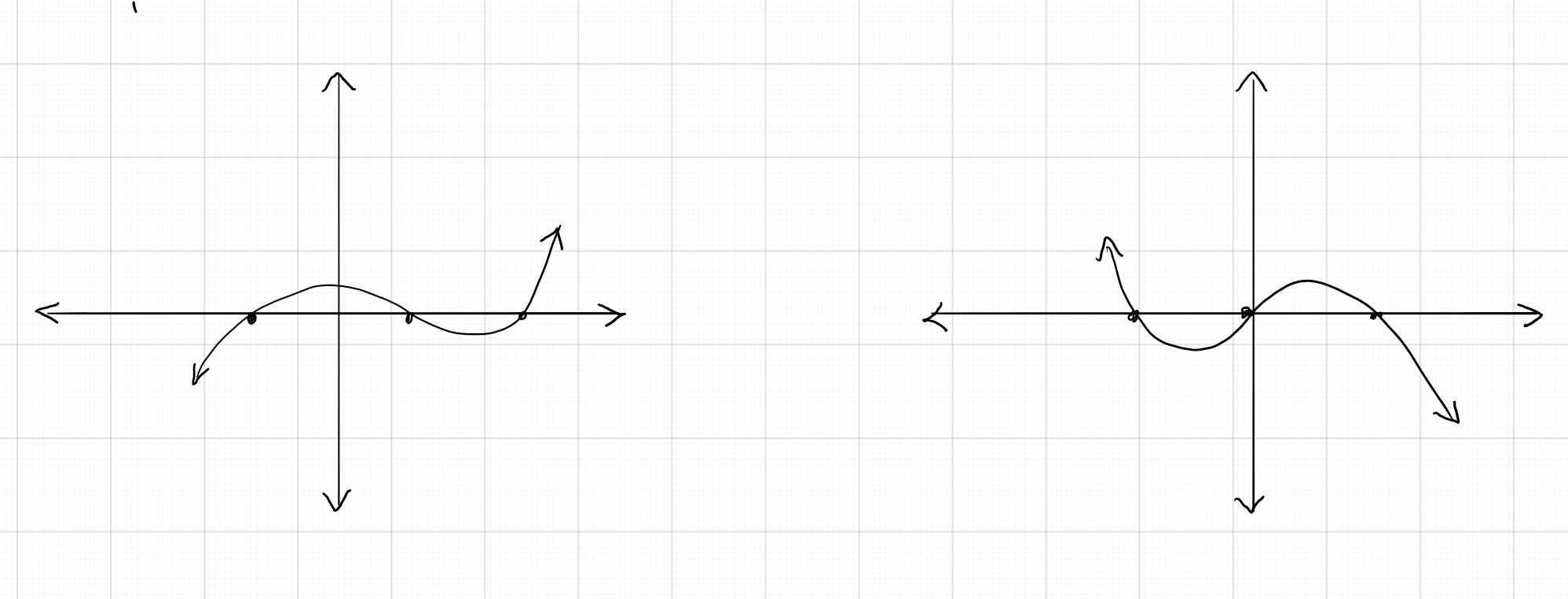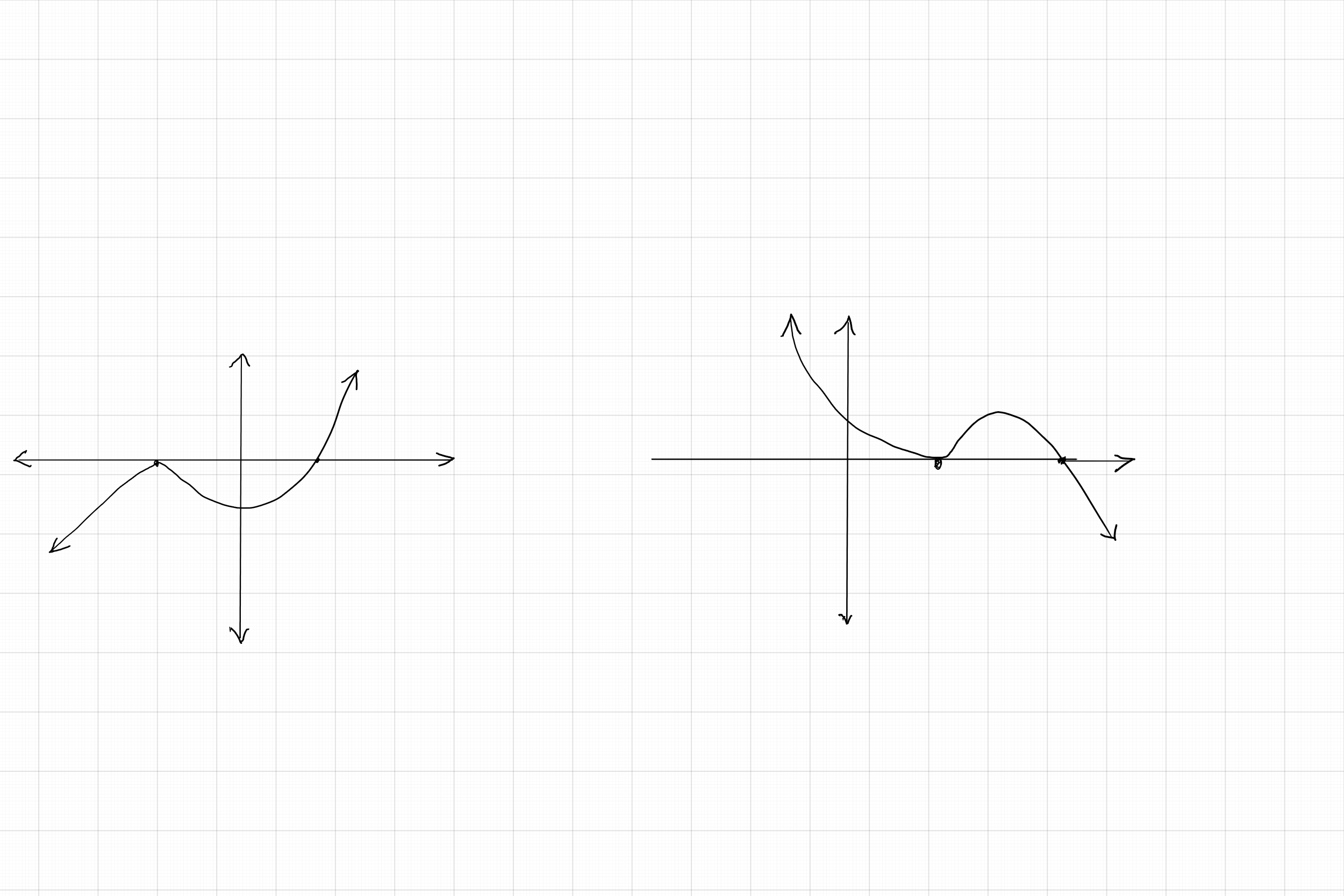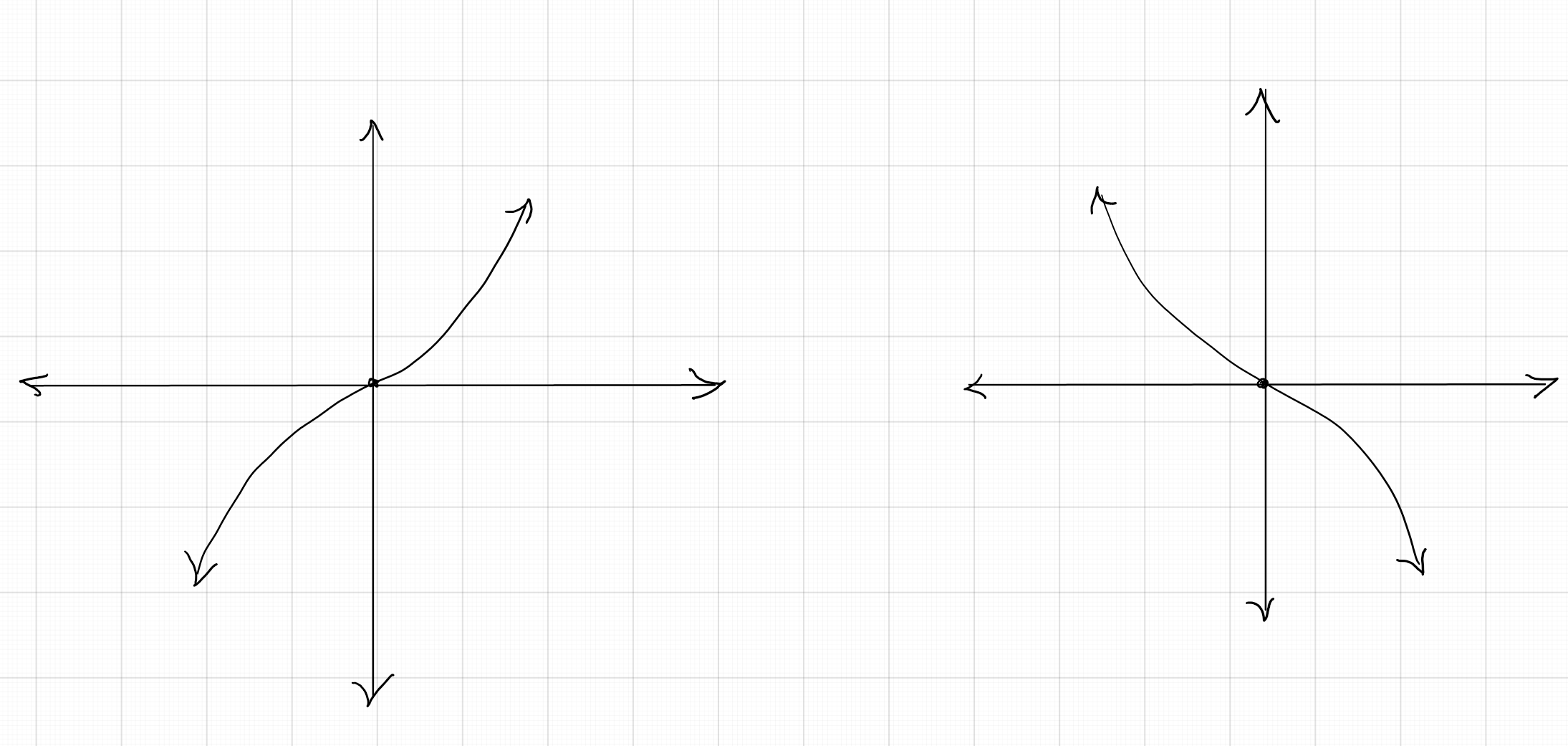## Practice Problems

Observe each of the graph given below and determine the number of distinct zeros of the polynomial whose graph is given.

$3$

$3$

$2$

$4$

$6$

### 2-2: Relationship Between the Zeros and Coefficients of a Polynomial

Consider a linear polynomial $p(x) = 2x + 5$. Then, its zero is obtained by setting $p(x) = 0$. That is, $2x + 5 = 0$. This gives $x = -\frac{5}{2}$. In general, zero of a linear polynomial $p(x) = ax+ b$ is $x = -\frac{b}{a}$, where $a$ is the coefficient of $x$ and $b$ is the constant term.

#### Example 1:

Find the zero of each of the following linear polynnomials: $(i) p(x) = 4x - 8 \quad (ii) p(x) = -3x + 1 \quad (iii) p(x) = 4x + 3$ without algebraically solving.

Solution:

$(i)$ In this problem, $a = 4$ and $b = -8$. So, if $\alpha$ is the zero of the polynomial, then, $\alpha =-\frac{-8}{4} = 2$
$(ii)$ Here, $a = -3$ and $b = 1$. So, the zero $\alpha = -\frac{1}{-3} = \frac{1}{3}$.
$(iii)$ Here, $a = 4$ and $b = 3$. So. the zero $\alpha = -\frac{4}{3}$

To understand the relationship between the zeroes and the coefficients of quadratic polynimials, we shall consider an example.

#### Example 2:

Find the zeroes of the quadratic polynomial $p(x) = 2x^2 - 5x + 3$

Solution:

Zeroes of this polynomial can be obtained by solving the equation $2x^2 - 5x + 3 = 0$. By the known factorizing method, we have product of the two zeroes $+6$ and sum $-5$. \begin{eqnarray} 2x^2 - 5x + 3 &=& 0\\ 2x^2 - 3x - 2x + 3 &=& 0\\ x(2x - 3) - 1(2x - 3) &=& 0\\ (x - 1)(2x - 3) &=& 0\\ \end{eqnarray}
zeroes of the polynomial are obtained by setting each factor to zero. If $\alpha$ and $\beta$ are the zeroes, then we have $\alpha = 1$ and $\beta = \frac{3}{2}$. Now, sum of the zeroes $\alpha + \beta = 1 + \frac{3}{2} = \frac{5}{2}$. Note that $-5$ is the coefficient of $x$ and $2$ is the coefficient of $x^2.$ Product of the zeroes $\alpha \beta = 1 \times \frac{3}{2} = \frac{3}{2}$. Note that the numerator $3$ is the constant term and the denominator $2$ is the coefficient of $x^2$.

Standard form of a quadratic polynomial is $p(x) = ax^2 + bx + c$. In general, if $\alpha$ and $\beta$ are the zeroes of this polynomial, then, the sum of the zeroes $\alpha + \beta = -\frac{b}{a}$, where $b$ is the coefficient of the $x$ term and $a$ is the coefficient of the $x^2$ term, i.e., the leading coefficient. Product of the zeroes $\alpha \beta = \frac{c}{a}$, where $c$ is the constant term and $a$ is the leading coefficient.

#### Example 2:

Find the zeroes of the polynomial $x^2 - 6x + 8$ and verify the relationship between the zeroes and the coefficients of the polynomial.

Solution:

First, let us solve the equation $x^2 - 6x + 8 = 0$ to find the zeroes: \begin{eqnarray} x^2 - 6x + 8 &=& 0\\ x^2 - 4x - 2x + 8 &=& 0\\ x(x - 4) -2(x - 4) &=& 0\\ (x - 4)(x - 2) &=& 0\\ \end{eqnarray}
Sum of the zeroes = $4 + 2 = 6 = \frac{6}{1}$. Coefficient of $x$ is $-6$ and coefficient of $x^2$ is $1$. Product of the zeroes is $(4)(2) = 8 = \frac{8}{1}.$ Constant term is $8$ and coefficient of $x^2$ is $1$. Thus the relationship is verified.

#### Example 3:

Find a quadratic polynomial such that the sum and product of its zeroes are $8$ and $15$ respectively.

Solution:

The standard form of a quadratic polynomial is $ax^2 + bx + c$. Let its zeroes be $\alpha$ and $\beta$. Then, according to the given information, $\alpha + \beta = -\frac{b}{a} = 8$ and $\alpha\beta = \frac{c}{a} = 15$. If $a = 1$, then, we get $b = - 8$ and $c = 15$. So, one polynomial is $x^2 - 8x + 15$. Generally, any quadratic polynomial of the form $k(x^2 - 8x + 15)$ would satisfy the given condition.

Standard form of a cubic polynomial is $p(x) = ax^3 + bx^2 + cx + d$. If $\alpha$, $\beta$, and $\gamma$ are zeroes of the cubic polynomial, then we have: \begin{eqnarray} \alpha + \beta + \gamma &=& -\frac{b}{a}\\ \alpha \beta + \beta \gamma + \alpha \gamma &=& \frac{c}{a}\\ \alpha \beta \gamma &=& -\frac{d}{a}\\ \end{eqnarray}

#### Example 4:

verify that $-1$, $2$, and $5$ are zeroes of the cubic polynomial $x^3 - 6x^2 + 3x + 10$. Then, verify the relationship between the zeroes and the coeffiients of the polynomial.

Solution:

Given that $-1$, $2$ and $5$ are zeroes of the cubic polynomial. This means that $x + 1$, $x - 2$, and $x - 5$ are factors of the polynomial. So, we need to just verify whether the product of these three factors results in the given polynomial: \begin{eqnarray} (x+1)(x - 2)(x - 5) &=& (x + 1)(x^2 - 7x + 10)\\ &=& x^3 + x^2 - 7x^2 - 7x + 10x + 10\\ &=& x^3 - 6x^2 + 3x + 10\\ \end{eqnarray}
Thus, we verify that the given numbers are zeroes of the given polynomial. Now, we verify the relationship between the zeroes and the coefficients: \begin{eqnarray} -1 + 2 + 5 &=& 6 = -\frac{b}{a}\\ (-1)(2) + (2 )(5) + (5)(-1) &=& 3 = \frac{c}{a}\\ (-1)(2)(5) &=& - 10 = -\frac{d}{a}\\ \end{eqnarray}
We also note that $a = 1$, $= - 6$, $c = 3$, and $d = 10$, thus verifying the relationship between the zeroes and the coefficients.

#### Example 5:

Find a cubic polynomial with leading coefficient $2$ that has $1$, $-3$, and $\frac{1}{2}$ as zeroes, using the relationship between the zeroes and the coefficients of the polynomial.

Solution:

Let us assume that $\alpha = 1$, $\beta = - 3$, and $\gamma = \frac{1}{2}$. Then, we have \begin{eqnarray} \alpha + \beta + \gamma &=& 1 - 3 + \frac{1}{2}\\ &=& \frac{2 - 6 + 1}{2}\\ &=& -\frac{3}{2}\\ \alpha\beta + \beta\gamma + \gamma\alpha &=& (1)(-3) + (-3)(\frac{1}{2}) + (\frac{1}{2})(1)\\ &=& -3 - \frac{3}{2} + \frac{1}{2}\\ &=& \frac{-6- 3 + 1}{2}\\ &=& -\frac{8}{2}\\ \alpha\beta\gamma &=& (1)(-3)(\frac{1}{2})\\ &=& -\frac{3}{2}\\ \end{eqnarray}
Foom the above computation, we have \begin{eqnarray} \alpha + \beta + \gamma &=& -\frac{3}{2} = -\frac{b}{a}\\ \alpha\beta + \beta\gamma + \gamma\alpha &=& -\frac{8}{2} = \frac{c}{a}\\ \alpha\beta\gamma &=& -\frac{3}{2} = -\frac{d}{a}\\ \end{eqnarray}
If $a = 2$, then we have $b = 3$, $c = - 8$, and $d = 3$. So, the required cubic polynomial is $2x^3 + 3x^2 - 8x + 3$.

## Practice Problems

For problems #1- 5, verify the relationship between the zeroes and the coefficients of each of the given quadratic polynomials:

Zeroes are $-4$ and $-2$.

Zeros are $4$ and $-2$.

Zeroes are $1$ and $-4$.

Only one zero $-3$ with multiplicity $2$.

Zeroes are $1$ and $-\frac{7}{3}$

For problems # 6 - 10, find a quadratic polynomial that has the given zeros:

$x^2 + x - 20$

$x^2 - 2$

$2x^2 + 3x - 2$

$6x^2 - x - 2$

$4x^2 + 3x - 1$

For problems # 11 - 15, verify that the given numbers are the zeros of each of the cubic polynomial . Then verfiy the relationship between the zeroes and the coefficients:

To verify.

To verify

To verify

To verify

To verify

For problems # 16 - 20, find a cubic polynomial that has the given zeroes:

$x^3+ 5x^2 - 12x - 36$

$x^3 + x^2 - 12x$

$2x^2 + 5x^2- 22x + 15$

$x^3 - 2x^2 - 3x + 6$

$x^3 - 4x^2 + 5x - 2$

### 2-3: Division Algorithm fof Polynomials

The division algorithm for real numbers that we learnt in earlier section can be extended to polynomials. The statement is as follows: If $A(x)$ and $B(x)$ are any two polynomials with $B(x) \neq 0$, Then, we can find two polynomials such that $A(x) = B(x) \times q(x) + r(x)$, where the degree of $r(x)$ is less than the degree of $B(x)$, or $r(x) = 0$.
Basically this means that, when $A(x)$ is divided by $B(x)$, you get a quotient $q(x)$ and a remainder $r(x)$, then, the rule that Dividend = Divisor x Quotient + Remainder.

#### Example 1:

Verify the division algorithm by dividing the polynomial $x^4 + 3x^3 - 3x^2 + 4x + 3$ by the polynomial $x^2 + x - 2$

Solution:

From the division process is shown below, we note that the quotient is $x^2 + 2x - 3$ and the remainder is $11x - 3$. According to the division algorithm, Didivend = Divisor x Quotient + Remainder. We shall verify this as below: \begin{eqnarray} x^4 + 3x^3 - 3x^2 + 4x + 3 &=& (x^2 + x - 2)(x^2 + 2x - 3) + (11x - 3)\\ &=& x^4 + 2x^3 - 3x^2 + x^3+ 2x^2 - 3x - 2x^2 - 4x + 6 + 11x - 3\\ &=& x^4 + (2x^3 + x^3) + (-3x^2 + 2x^2 - 2x^2) + (-3x - 4x + 11x) + (6 - 3)\\ &=& x^4 3x^3 - 3x^2 + 4x + 3\\ \end{eqnarray}
Thus, division algorithm is verified.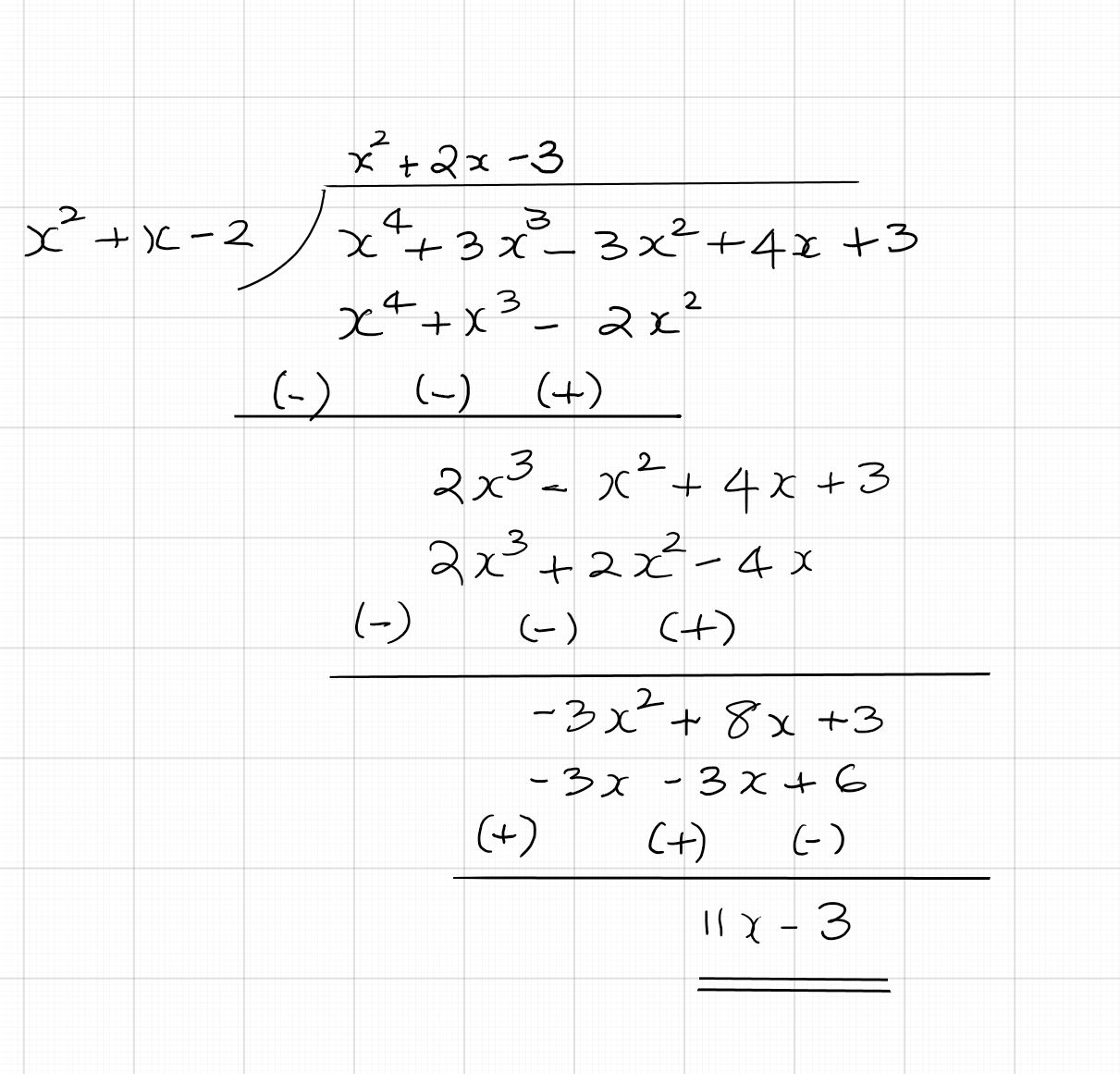'

Division algorithm can be used to find the zeroes of a polynomial, is some of the zeroes are given, as illustrated in the following example.

#### Example 2:

Find all the zeroes of the polynomial $2x^4 + 2x^3 - 13x^2 - x + 6$, given that $\frac{1}{\sqrt{2}}$ and $-\frac{1}{\sqrt{2}}$ are two of the zeroes.

Solution:

Since $\frac{1}{\sqrt{2}}$ and $-\frac{1}{\sqrt{2}}$ are zeroes of the polynomial, $\left(x - \frac{1}{\sqrt{2}}\right)\left(x + \frac{1}{\sqrt{2}}\right) = x^2 - \frac{1}{2}$ is a factor of the polynomial. That is, $\frac{1}{2}(x^2 - 1)$ is a factor. Now, we divide the given polynomial by $x^2 - 1$ as shown below: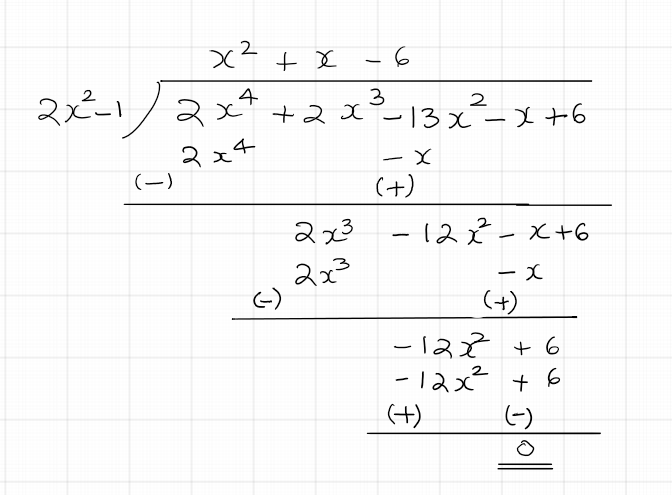From the division shown above, we have quotient = $x^2 + x - 6$. As the divisor is a factor of the dividend, we get the remainder $0$. Factorizing the quotient, we get $x^2 + x - 6 = (x - 2)(x + 3)$. Setting each of these factors to zero gives us the other two zeroes of the polynomial as $2$ and $-3$. So, the zeroes of the given polynomial are $2$, $-3$, $\frac{1}{\sqrt{2}}$ and $-\frac{1}{\sqrt{2}}$

The example below shows use of division algorithm in solving another type of problem:

#### Example 3:

On dividing the polynomial $x^4 - 2x^3 + x^2 + 3x - 8$ by a polynomial $g(x)$, the quotient is $x^2 - 4x + 12$ and the remainder is $-33x + 28$. Find $g(x)$.

Solution:

Using the division algorithm and substituting for the dividend, quotient, and remainder, we can solve for the divisor $g(x)$. We have $x^4 - 2x^3 + x^2 + 3x - 8 = (x^2 - 4x + 12)[g(x)] + (-33x + 28)$. Subtracting the remainder from both sides gives $x^4 - 2x^3 + x^2 + 36x - 36$. This means that $x^2 - 4x + 12$ and $g(x)$ are factors of the given polynomial. Hence, we can get $g(x)$ by dividing the given polynomial by $x^2 - 4x + 12$: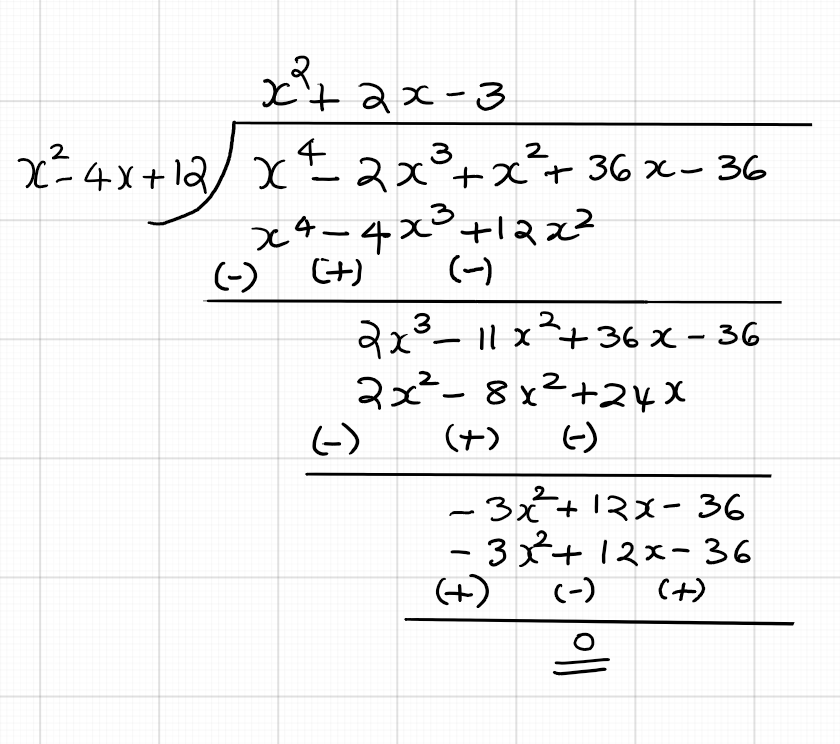## Practice Problems

For problems # 1 - 4, divide each polynomial $p(x)$ by the polynomial $d(x)$ and find the quotient and remainder .

Quotient is $x^2$ and remainder is $5$.

Quotient is $x - 6$ and remainder is $-6x + 2$

Quotient is $x + 2$ and remainder is $-x + 11$.

Quotient is $x^2 + x + 1$ and remainder is $2x + 9$.

No. Because, we get a non-zero remainder upon division.

Yes. we get a zero remainder upon division.

$-4$

$3$ and $1$ are the other zeroes.

$d(x) = x^2 - 2x + 3$.

$d(x) = x^2 + x - 2$